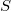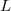﻿

### Representation growth and representation zeta functions of groups

#### Abstract

We give a short introduction to the subject of representation growth and representation zeta functions of groups, omitting all proofs. Our focus is on results which are relevant to the study of arithmetic groups in semisimple algebraic groups, such as the group$S$$L$$n$ (Z) consisting of n x n integer matrices of determinant 1 . In the last two sections we state several results which were recently obtained in joint work with N. Avni, U.Onn and C.Voll.

DOI Code: 10.1285/i15900932v33n1p107

Keywords: Representations; characters; arithmetic groups; p-adic Lie groups; zeta functions

Full Text: PDF